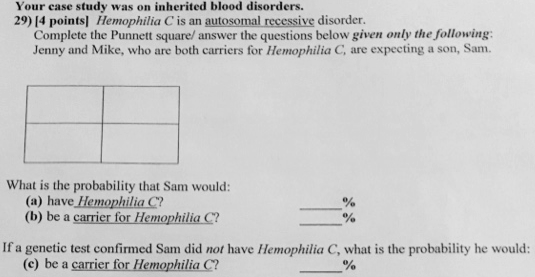# Problem: Hemophilia C is an autosomal recessive disorder. Complete the Punnet square and answer the questions below, given only the following:Jenny and Mike, who are both carriers for Hemophilia C, are expecting a son, Sam.a. What is the probability that Sam would have Hemophilia C?b. What is the probability that Sam would be a carrier for Hemophilia C?If a genetic test confirmed Sam did not have Hemophilia C, what is the probability he would c. be a carrier for Hemophilia C?

###### FREE Expert Solution

The trait in question is stated to be autosomal recessive. This means that Sam, the couple's future son, need to have two copies of the recessive allele to be afflicted.###### Problem Details

Hemophilia C is an autosomal recessive disorder. Complete the Punnet square and answer the questions below, given only the following:

Jenny and Mike, who are both carriers for Hemophilia C, are expecting a son, Sam.

a. What is the probability that Sam would have Hemophilia C?

b. What is the probability that Sam would be a carrier for Hemophilia C?

If a genetic test confirmed Sam did not have Hemophilia C, what is the probability he would

c. be a carrier for Hemophilia C?